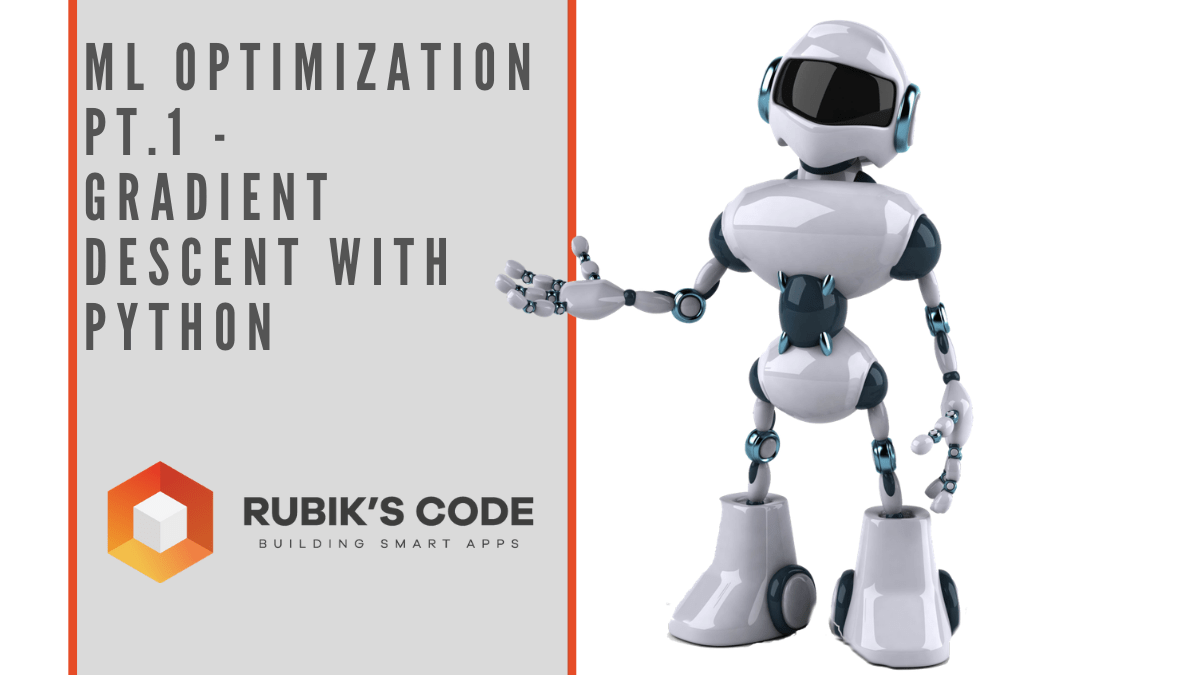Select Page## ML Optimization pt.1 – Gradient Descent with Python

The code that accompanies this article can be found here. So far in our journey through the Machine Learning universe, we covered several big topics. We investigated some regression algorithms, classification algorithms and algorithms that can be used for both types...## 14 Popular Machine Learning Evaluation Metrics

The code that accompanies this article can be found here. Thus far in our journey through Machine Learning Basics, we covered several topics. We investigated some regression algorithms, classification algorithms and algorithms that can be used for both types of...## Back to Machine Learning Basics – Regularization

The code that accompanies this article can be found here. In a previous couple of articles, we explored some basic machine learning algorithms. Thus far we covered some simple regression algorithms, classification algorithms and algorithms that can be used for both...## Back to Machine Learning Basics – Clustering

The code that accompanies this article can be found here. In a previous couple of articles, we explored some basic machine learning algorithms. Thus far we covered some simple regression algorithms, classification algorithms and we started with algorithms that can be...## Back to Machine Learning Basics – Decision Tree & Random Forest

The code that accompanies this article can be found here. In a previous couple of articles, we explored some basic machine learning algorithms. Thus far we covered some simple regression algorithms, classification algorithms and we started with algorithms that can be...## Back to Machine Learning Basics – Support Vector Machines

The code that accompanies this article can be found here. In a previous couple of articles, we started exploring some of the basic machine learning algorithms. We covered some simple regression and classification algorithms. We also used other technologies like...